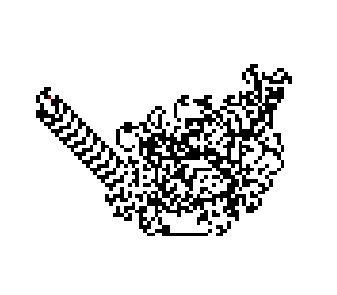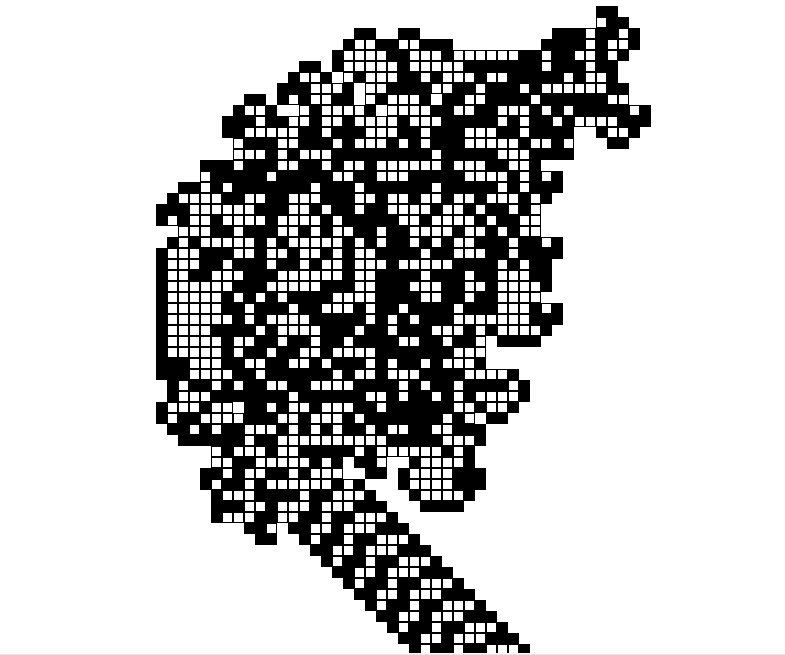# Python | Langton’s Ant

Langton’s Ant is a 4-state two-dimensional universal Turing machine. It was invented by Chris Langton in 1986. It is basically an ant, sitting on a square lattice of cells, which are initially white. The ant moves on the plane and changes the color of cells creating patterns on it. But the movement of the ant is not random; it follows the following set of rules :

• If the ant is on a black square, it turns right 90 degrees and moves forward one unit.
• If the ant is on a white square, it turns left 90 degrees and moves forward one unit.
• When the ant leaves a square, it inverts the color.

As the ant starts, it creates a black and white pattern while moving. Initially, the changes are not distinctive but as we iterate it over and over again, a beautiful pattern emerges. But if we further increase the number of iterations (say ~ 10000), the ant starts repeating its path with a gradual shift, instead of making new patterns. Thus, we obtain a highway like pattern that is infinite. The ant keeps moving on that highway and gives the following pattern.Refer the visual explanation of Langton’s Ant from here. It helps to visualize how exactly the ant works.

The Python-3 code for Langton’s Ant is given below :

 `# importing turtle module ` `import` `turtle ` ` `  `def` `langton(): ` ` `  `    ``# Initializing the Window ` `    ``window ``=` `turtle.Screen() ` `    ``window.bgcolor(``'white'``) ` `    ``window.screensize(``1000``,``1000``) ` ` `  `    ``# Contains the coordinate and colour ` `    ``maps ``=` `{} ` ` `  `    ``# Initializing the Ant ` `    ``ant ``=` `turtle.Turtle() ` `     `  `    ``# shape of the ant ` `    ``ant.shape(``'square'``)     ` `     `  `    ``# size of the ant ` `    ``ant.shapesize(``0.5``) ` `     `  `    ``# speed of the ant ` `    ``ant.speed(``10000``)                                  ` `     `  `    ``# gives the coordinate of the ant                 ` `    ``pos ``=` `coordinate(ant)                              ` `     `  `    ``while` `True``: ` `         `  `        ``# distance the ant will move ` `        ``step ``=` `10`                                      `        ``if` `pos ``not` `in` `maps ``or` `maps[pos] ``=``=` `"white"``: ` `             `  `            ``#inverts the colour ` `            ``ant.fillcolor(``"black"``)         ` `             `  `            ``#stamps a copy of the ant on the canvas ` `            ``ant.stamp()                                  ` `            ``invert(maps, ant, ``"black"``) ` `            ``ant.right(``90``) ` `             `  `            ``#moves the ant forward ` `            ``ant.forward(step)                          ` `            ``pos ``=` `coordinate(ant) ` `             `  `        ``elif` `maps[pos] ``=``=` `"black"``: ` `            ``ant.fillcolor(``"white"``) ` `            ``invert(maps, ant, ``"white"``) ` `             `  `            ``ant.stamp() ` `            ``ant.left(``90``) ` `            ``ant.forward(step) ` `            ``pos ``=` `coordinate(ant) ` ` `  `def` `invert(graph, ant, color): ` `    ``graph[coordinate(ant)] ``=` `color ` ` `  `def` `coordinate(ant): ` `    ``return` `(``round``(ant.xcor()), ``round``(ant.ycor())) ` ` `  `langton() `

Output :Here, the white cells are denoted by ‘ ‘ (space) and the black cells are denoted by ‘•’ (dot).

My Personal Notes arrow_drop_upCheck out this Author's contributed articles.

If you like GeeksforGeeks and would like to contribute, you can also write an article using contribute.geeksforgeeks.org or mail your article to contribute@geeksforgeeks.org. See your article appearing on the GeeksforGeeks main page and help other Geeks.

Please Improve this article if you find anything incorrect by clicking on the "Improve Article" button below.

Article Tags :

1

Please write to us at contribute@geeksforgeeks.org to report any issue with the above content.